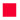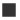# Glossary

- Basic Knowledge of  Thermistors -Basic KnowledgeSelection GuideApplication ExampleGlossaryDiscontinued Products Information
 Term Definition Curie Point The resistance of the POSISTOR remains almost constant up to a certain temperature, and the resistance suddenly increases after a certain temperature. The changing point of this resistance is called the "Curie point (Curie temperature) ", and Murata Manufacturing defines this point as the temperature where the resistance becomes double of the resistance at 25°C. Temperature Compensation Temperature compensation is to adjust the temperature differential caused by temperature changes in instruments, measuring devices etc., by using specially designed attachments and electrical circuits. This is to suppress the variations in the temperature change in components where their characteristics vary by temperature. Inrush Current This is a large current which flows beyond a steady state current which occurs when the power supply of electronic equipment is turned on. Positive Temperature Coefficient Thermistor The characteristic which increases the resistance when the temperature is increased is called the positive characteristic, and the temperature characteristic of the PTC Thermistor is the positive characteristic. For this reason, it is also called a Positive Temperature Coefficient Thermistor. Negative Temperature Coefficient Thermistor The reverse characteristic where the resistance decreases when the temperature is increased is called the negative characteristic, and the temperature characteristic of the NTC Thermistor is the negative characteristic. For this reason, the NTC Thermistor is also called a Negative Temperature Coefficient Thermistor. B Constant The constant which shows the change in the resistance calculated by the following formula using the resistance at 2 points at a specified ambient temperature. B=ln (R/R0) / (1/T-1/T0) R: Resistance when ambient temperature is T(K), R0: resistance when the ambient temperature is T0 (K) Maximum Operating Voltage Refers to the maximum voltage which can be applied constantly to a POSISTOR within an operating temperature range. Withstand Voltage Refers to the voltage which a POSISTOR can withstand when a voltage is applied for 3 minutes in still air at 25°C. A method to apply a voltage gradually from 0V to the withstand voltage is used. Heat Dissipation Coefficient (D) Refers to the amount of heat which is lost over a unit of time, based on a 1°C temperature difference between the heating element and ambient temperature. W=I・V=D (T-T0) T: Heating element temperature To: mbient temperature D. : Heat dissipation factor (W/°C) These values are generally determined by the dimensions, structure and material of the heating element. Thermal Time Constant (γsec) The thermal time constant is expressed by the time to reach 0.632 times the temperature difference when the POSISTOR is instantly moved in an ambient temperature from T0 to T1. Generally, this is expressed by γ=H/D from the heat dissipation factor (W/°C) and the heat capacity H (W・sec/°C). This is related to the dynamic characteristic. Operating Point The operating point where the self-heating of a POSISTOR and the heat dissipation to outside became equilibrium states. Protection Current The maximum point of the current in the current and voltage characteristics of a POSISTOR, is called the protection current. Protection Current Fluctuation Range The protection current of a POSISTOR fluctuates by the ambient temperature, resistance, temperature characteristics, shape and etc. The current range beyond the upper limit of the protection current is called the operating range, and the current range below the lower limit is the non-operating current range, and the current range between the upper limit and lower limit is called the protection current fluctuation range. Operating Time The operating time refers to the period until the inrush current flowing into the POSISTOR is decreased by 1/2.Basic KnowledgeSelection GuideApplication ExampleGlossaryDiscontinued Products Information